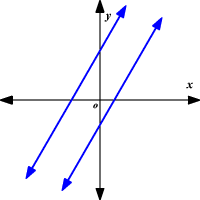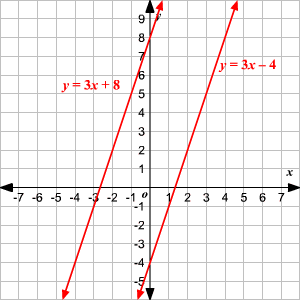# Parallel Lines

Parallel lines are coplanar lines that do not intersect. In two dimensions, parallel lines have the same slope .Example:

The two lines

$\begin{array}{l}y=3x+8\\ y=3x-4\end{array}$

are parallel, since they both have slope $3$ .